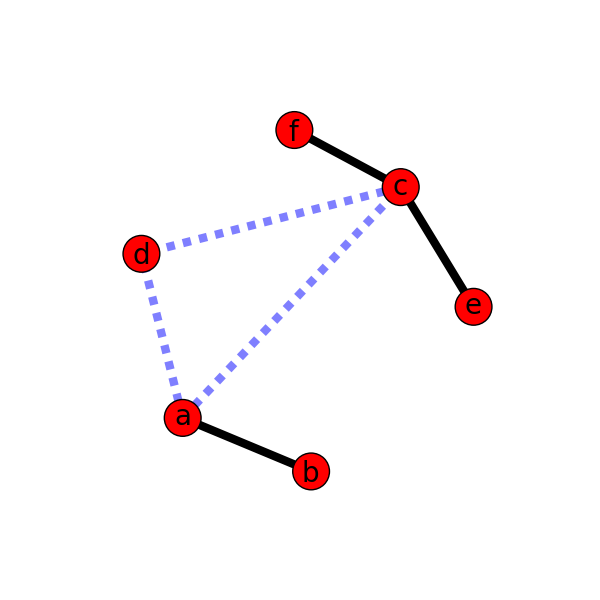Warning

This documents an unmaintained version of NetworkX. Please upgrade to a maintained version and see the current NetworkX documentation.

# Weighted Graph¶#!/usr/bin/env python
"""
An example using Graph as a weighted network.
"""
__author__ = """Aric Hagberg (hagberg@lanl.gov)"""
try:
import matplotlib.pyplot as plt
except:
raise

import networkx as nx

G=nx.Graph()

elarge=[(u,v) for (u,v,d) in G.edges(data=True) if d['weight'] >0.5]
esmall=[(u,v) for (u,v,d) in G.edges(data=True) if d['weight'] <=0.5]

pos=nx.spring_layout(G) # positions for all nodes

# nodes
nx.draw_networkx_nodes(G,pos,node_size=700)

# edges
nx.draw_networkx_edges(G,pos,edgelist=elarge,
width=6)
nx.draw_networkx_edges(G,pos,edgelist=esmall,
width=6,alpha=0.5,edge_color='b',style='dashed')

# labels
nx.draw_networkx_labels(G,pos,font_size=20,font_family='sans-serif')

plt.axis('off')
plt.savefig("weighted_graph.png") # save as png
plt.show() # display